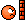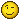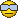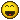Welcome to WSL!

Make yourself at home, but before posting, please may I ask you to read the following topics.

Posting 101
Server space, screenshots, and you

Thank you!## Interpolation functions in Fuses?

pixelstuff
Posts: 46
Joined: Sun Jul 15, 2018 11:50 am
Been thanked: 1 time

### Interpolation functions in Fuses?

Does anyone know if its possible to interpolate a new set of positions as a function in a .fuse, there is a command to convert to a bspline on masks but nothing as far as I can see in the docs or any of the examples in the shape fuses. I have looked into interpolation algorithms(linear seems the easiest) but in .lua that is still difficult.Code: Select all

``````-- Lines to points
local line1 = Shape()
line1:MoveTo(start1.X, convertY(start1.Y, img))
line1:LineTo(V3,convertY(V3,img)) -- Line to Mid
line1:LineTo(P2,convertY(P2,img)) -- Line to end``````
I have 3 points I need to interpolate in some way which ever way is easiest, hopefully just something simple. If its not simple, then this maybe a possibility

De Casteljau's algorithm

Or
Catmull-rom interpolation
https://github.com/pkulchenko/ZeroBrane ... ullrom.lua

I looked into math.cos() and math.sin which is how most of the circular shapes are created, but would give a perfect arc which isn't what I want.

I would need the current Middle Vector point I have to act as a Bspline control for a new interpolated(curved) set of points that use the original 3 points as reference.

Here is a loop I would use to plot the new points and lines(currently just a circle) but I hopefully will be able to interpolate the points and resample using "sides" number

Code: Select all

``````line2:MoveTo(start1.X, convertY(start1.Y, img))
for i = 1, sides - 1 do

line2:LineTo(P1+cos(i/sides * math.pi * 2 + angle) * r,convertY(P1+sin(i/sides * math.pi * 2 + angle) * r,img))
end``````

Best solution would be Shape() = MakeItSmooth(How.Smooth?)SirEdric
Fusionator
Posts: 2310
Joined: Tue Aug 05, 2014 10:04 am
Real name: Eric Westphal
Been thanked: 42 times
Contact:

### Re: Interpolation functions in Fuses?

Hi Pixelstuff.

Those interpolations in Fusion are driven by the position of the handles (which you could set along with the KeyFrames).
So you would need to calculate those.
Or (if we're talking animation BezierSplines) you could have a look here: viewtopic.php?f=6&t=2622

Midgardsormr
Fusionator
Posts: 1757
Joined: Wed Nov 26, 2014 8:04 pm
Location: Los Angeles, CA, USA
Been thanked: 89 times
Contact:

### Re: Interpolation functions in Fuses?

I think we're actually talking vector drawing with a Fuse, not manipulation of Fusion's existing splines. Is that right? This is a problem I've wanted to solve for a while, since I learned that the built-in BezierTo() function is broken. I'm very pleased to see someone else taking it on!

The simplest method I can think of would be to use a sub-division approach, where you're using a loop to repeatedly call LineTo(). This will create a BSpline-like spline, where the line won't pass through the control points. This has the advantage of letting you use Fusion's existing Shape() functions to render the spline. It's the method I've been looking at to create a "fractalizer" that might make better-looking lightning than the existing Lightning Fuse.

Or, you could create your own spline function, where you can choose exactly which algorithm you want to use, including the Bezier, which does pass through the control point, as illustrated in your graph. You'd have much more control over the shape, but you'd also have to write your own rendering routines, since you wouldn't be able to plug it into Shape().

It might also be possible to use a sort of hybrid of the two, where you use your custom spline function to determine the end-points of a set of LineTo() calls. That would get you the best of both worlds, but it also means solving both of the previous problems to get there.

I mentioned the Lightning Fuse above. It might be a good place to look to see how to create chained LineTo() calls, which is similar to what you'll need to do:
https://www.steakunderwater.com/VFXPedi ... _v1_1.Fuse

It's been a long time since I looked at it, though. Not sure how much help it will be.

pixelstuff
Posts: 46
Joined: Sun Jul 15, 2018 11:50 am
Been thanked: 1 time

### Re: Interpolation functions in Fuses?Thanks both for your help, yes its just using lineto() to create the lines in the fuse. Some vectors and variables setup for this but they can get really confusing really fast when things get complicated.

I will try and see if I can your nested lineto loop to work. I have created a for loop that creates multiple segments from the bottom 2 points, then tried to use the rotated vector to position them in a curve but I can't get the bottom point distance value to ramp the normalized vector up and down to create the shape.

Another idea is the bottom solution, divide up the positions on one side > get the distance > then rotate and position each vector. That will probably drive me nuts trying and I have little control over the curve.

I have only just found this bit of code, that may come in useful, I just don't understand how it works yet.

Bezier Interpolation(it could be good to have control over the shape as it would use handles)

Code: Select all

``````--a line from x1, y1 to x2, y2 as t varies from 0 to 1
function line(x1, y1, x2, y2)
return function(t)
return x1 + (x2 - x1) * t, y1 + (y2 - y1) * t
end
end

--the Bezier curve formed by interpolating between multiple given curves or lines
function curve(c1, c2, c3, ...)

if not c2 then return c1 end    --sanity check

local c1c2 = function(t)
local x1, y1 = c1(t)
return line(x1, y1, c2(t))(t)
end

return c3 and curve(c1c2, curve(c2, c3, ...)) or c1c2
end

--the Bezier curve determined by given control points
function bezier(xv, yv)
lines = {}
for i = 1, #xv-1 do
lines[i] = line(xv[i], yv[i], xv[i+1], yv[i+1])
end
return curve(unpack(lines)) or lines
end

``````
In Houdini(VEX) you would just use

spline("catrom", t, @P, v1, v2, v3)

to return the position

It uses 3 points to calculate the cat-mull rom interpolation and t is just the offset, but in Lua you would need to manually write that function.

Midgardsormr
Fusionator
Posts: 1757
Joined: Wed Nov 26, 2014 8:04 pm
Location: Los Angeles, CA, USA
Been thanked: 89 times
Contact:

### Re: Interpolation functions in Fuses?

I unfortunately don't have the time to really dig into it at the moment, but I think a recursive function similar to this would be useful:

Code: Select all

``````subdivide(x1,y1, x2,y2, x3,y3, subdivs)
-- Points 1 and 2 are the end points of the curve, and x3 is a control point in the middle, creating two line segments.
-- Subdivs is the number of iterations to perform
i = 0
if i < subdivs then
-- Calculate midpoints.
xa = (x3 - x1) / 2
ya = (y3 - y1) / 2
xb = (x2 - x3) / 2
yb = (y2 - y3) / 2
-- These two ordered pairs, a and b, describe a new line segment, each end of which forms a new linear curve that is the
-- basis for another subdivision
subdivide(x1,y1, xb,yb, xa,ya, subdivs - 1)
subdivide(xa,ya, x2,y2, xb,yb, subdivs - 1)
else -- Draw a line segment
segment = Shape()
segment:MoveTo(x1, y1)
segment:LineTo(x2, y2)
end
end
``````

I'm not sure how close that is to something useful, but it's roughly the algorithm I was working on a few months ago, and it's close to the process described in the Khan Academy course, if I recall correctly.

Fusion is supposed to have a function that does this, but as I said before, it's broken.

pixelstuff
Posts: 46
Joined: Sun Jul 15, 2018 11:50 am
Been thanked: 1 time

### Re: Interpolation functions in Fuses?

Thank you, hopefully they will fix that function, but on the up side you may also have some more control this way by building it from the ground up.

Your recursive function was going in the same direction to the version I have now. I think that your recursion idea would be very good for when you need a flexible amount of points for the guide points. The bit where you divided up the edges was correct, and in line with what I did. So I thought I would share some process.

I was getting a little bit lost in bits of code I found from different places. So I went back to understanding the concepts and found a good example of how the Casteljau's algorithm works as soon as I worked out the motion of the middle point it started to all make sense.

A quick illustration I did to visualize the concept
--

If you treat the points as rails that slide up and down the right and left edges and the middle point that slides left to right, it creates the motion needed.

So going from 0-1 left to right with the mid points that are offset this drives the position of the middle point on the middle segment and its position left to right along the "rail", that's the best way to think of it.

The direction of the vectors is really important so that all of the points move correctly at the same time(not easy to visually check) so I had to brute force a line to each one of the vector positions of the points to make sure it was all working correctly.

In a nut shell the steps would be

1) Define vectors for the reference points
2) Create the middle points on the rails you can use as many as you need to but I used just a few, as this gets complicated really quickly
3) You need an offset value to move all of the points along the "rails", this then becomes the length of each line segment(do the re-sampling once you have it working)
4) Work out all of the vectors driving the points
5) Re sample the segments 1.0 is the total length / number of sides
6) Do some of this-

Code: Select all

``````	 -----------------

-- P1 is left bottom point X P1 is left bottom point Y
-- P2 is Right bottom point X P2 is Right bottom point Y
-- V3 is offset middle point X V3 is offset middle point Y

local line2 = Shape()
step = 0 -- Number off segments
step = 1/sides -- 1 is the total length / sides resamples the curve
slide = 0
line2:MoveTo(P1, P1, img) -- Start Position

for i = 1,sides,1 -- Loop to slide middle point around
do
slide = slide+step -- Length of each segment
LM = {((V3-P1)*slide)+P1,((V3-P1)*slide)+P1} -- Vector left middle
RM = {((P2-V3)*slide)+V3,((P2-V3)*slide)+V3} -- Vector right middle
MM = {((RM-LM)*slide)+LM,((RM-LM)*slide)+LM} -- Vector Middle middle

line2:LineTo(MM,convertY(MM,img)) -- draw line to End of segement
print("side: "..i) -- debug
print("slide: "..slide) -- debug
end

print("step: "..step) -- debug
print("--------------") -- debug

line1:AddShape( line2:OutlineOfShape(thickness, outlinetypes[linetype], "OJT_Round", (req:IsQuick() and 8 or 16)) )

----------------------

``````

tberakis
Posts: 33
Joined: Mon Dec 29, 2014 2:30 pm
Been thanked: 2 times

### Re: Interpolation functions in Fuses?

If you are actually after catmul-rom, then maybe something like this would help, too:

Code: Select all

``````function catrom1d(t, p1, p2, p3, p4)
return 0.5 * ((2 * p2) + (-p1 + p3) * t + (2*p1 - 5*p2 + 4*p3 - p4) * t^2 + (-p1 + 3*p2 - 3*p3 + p4) * t^3)
end

function catrom2d(t, p1, p2, p3, p4)
return { catrom1d(t, p1, p2, p3, p4), catrom1d(t, p1, p2, p3, p4) }
end
``````
This is fairly standard catmul-rom spline interpolation, where the function takes 't', the interpolation position from 0.0 .. 1.0, and four points, where the middle two are the ones you are interpolating between and the first and last are previous/next. Catmul-rom goes "through" all points, so where t=0, you will get exactly p2, and where t=1, you will get p3, with appropriate interpolation for in between values.

Generally recursive approximation is used based on the delta between results, to avoid some line segments being a significantly larger step than others. It's not necessary for basic constant-step interpolation. So to do that, you can recursively use catrom (or your interpolation of choice) between two 't' values, check the line length, and subdivide if below a threshold, ensuring you don't get steppiness in some parts of a curve.

pixelstuff
Posts: 46
Joined: Sun Jul 15, 2018 11:50 am
Been thanked: 1 time

### Re: Interpolation functions in Fuses?

Thanks for sharing this, the Casteljau's algorithm works best for what I need with just the 3 control points that I have and means I don't need to create the extra "invisible" points that are used for catmul-rom. But what you have there would be great to iterate through a series of points, that would be easily update-able. This makes me question how the original polygon masks are created. They seem to be stored as a table of results positions, and handles that are relative to the points?

I'm not sure how you would access the code on a polygon mask, to see if the "lineto" iteration can be interrupted to then be able to animate the stroke along the path?

Dunn
Moderator
Posts: 493
Joined: Mon Aug 04, 2014 4:27 am
Location: Hamburg, Germany
Been thanked: 3 times
Contact:

### Re: Interpolation functions in Fuses?

I just dug out some old code i was once working on too. Never got to finish it ( Somehow the BezierTo is not working right )
I can get the point count on the shape but can't access the points themselves .. maybe one can help out ( cdata<struct Shape *>: )

donno.. maybe this can help.Screenshot 2019-01-09 at 23.22.40.png
You do not have the required permissions to view the files attached to this post.

Midgardsormr
Fusionator
Posts: 1757
Joined: Wed Nov 26, 2014 8:04 pm
Location: Los Angeles, CA, USA
Been thanked: 89 times
Contact:

### Re: Interpolation functions in Fuses?

I finally got around to tackling this thing again. Here's my implementation of De Casteljau's algorithm.
You do not have the required permissions to view the files attached to this post.
Last edited by Midgardsormr on Thu Aug 29, 2019 5:03 pm, edited 1 time in total.

Midgardsormr
Fusionator
Posts: 1757
Joined: Wed Nov 26, 2014 8:04 pm
Location: Los Angeles, CA, USA
Been thanked: 89 times
Contact:

### Re: Interpolation functions in Fuses?

Turns out BezierTo() is not, in fact, broken in Fusion 9. We were just feeding it the wrong data. It takes three tables and a Boolean:

`Shape:BezierTo( {x2, y2}, {x3, y3}, {x4, y4}, [closed] )`

{x1,y1} is implicit from the current end-point of the Shape, just like LineTo(). There doesn't seem to be a way to control the subdivision level for the curve, so it's very faceted, making me think that my own implementation is a little superior visually. Many thanks, once again, to Peter Loveday for the hints.

The next challenge is to find out if it's possible to read a Polyline. I've figured out how to build the control, but I can't yet read the data from it.
You do not have the required permissions to view the files attached to this post.

Dunn
Moderator
Posts: 493
Joined: Mon Aug 04, 2014 4:27 am
Location: Hamburg, Germany
Been thanked: 3 times
Contact:

### Re: Interpolation functions in Fuses?

Jeeez! Finally ! Thanks alot Bryan.I had given up on this function after lots of hair pulling. looking back, my first Shape/BezierTo project was in 2017ddddaaammnn!

Midgardsormr
Fusionator
Posts: 1757
Joined: Wed Nov 26, 2014 8:04 pm
Location: Los Angeles, CA, USA
Been thanked: 89 times
Contact:

### Re: Interpolation functions in Fuses?

Peter came to the rescue once again with an explanation of the FlatBezierTo() method. This one turns the Bezier into lines (flattening it), much like my brute-force method does. It has an additional parameter for precision:

`Shape:FlatBezierTo( {x2, y2}, {x3, y3}, {x4, y4}, precision, [closed] )`

He also provided some additional information:
Then there's FlattenOfShape(precision), which returns a new shape with all beziers/conics flattened (again default 8)

A few other functions take a flattening precision too. ExpandOfShape(amount, exp_precision, flatten_precision), OutlineOfShape(thickness, linetype, jointype, outline_precision, windingmode, flatten_precision)

FlatConicTo(p2, p3, precision, close)

I tried `FlattenOfShape()`, but it didn't seem to do anything. The precision parameter on `OutlineOfShape()` works. The available options for windingmode appear to be: `SWM_NoChange`, `SWM_Normal`, `SWM_Clear`, `SWM_Solid`.